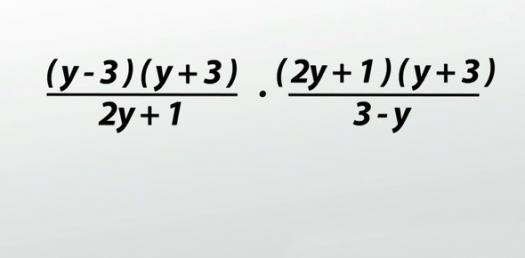Multiplying And Dividing Monomials

20 Questions | Total Attempts: 3251Settings• 1.
(x4)(x4)
• A.

X^4

• B.

X^8

• C.

X^6

• 2.
(-x3)(-x3)
• A.

-x^6

• B.

-x^9

• C.

X^6

• 3.
A2(a3)(a6)
• A.

A^11

• B.

A^9

• C.

A^5

• 4.
X(x2)(x7)
• A.

X^10

• B.

X^5

• C.

X^9

• 5.
(y2z)(yz2)
• A.

Yz^4

• B.

Y^3z^3

• C.

Y^2z^2

• 6.
(cd2)(c3d2)
• A.

C^3d^4

• B.

C^4d^4

• C.

Cd^4

• 7.
(4xy3)(3x3y5)
• A.

12x^4y^8

• B.

12xy^8

• C.

7x^4y^8

• 8.
(-5m3)(3m8)
• A.

-2m^11

• B.

-8m^11

• C.

-15m^11

• 9.
(102)3
• A.

10^6

• B.

20^3

• C.

5^5

• 10.
(e2f4)(e2f2)
• A.

Ef^10

• B.

E^2f^8

• C.

E^4f^6

• 11.
N9/n5
• A.

2n^6

• B.

N^9

• C.

N^4

• 12.
2n4/n
• A.

2n^3

• B.

2/n^3

• C.

N^4

• 13.
X3y4/x2y
• A.

-4x^3

• B.

-4y^7

• C.

Xy^3

• 14.
20x3y8/-5x3y
• A.

-4x^3

• B.

-4y^7

• C.

-4y

• 15.
3a5b2/9a2b5
• A.

5ab^8

• B.

15a^2

• C.

A^3/3b^3

• 16.
30a9b2/2a6b2
• A.

15a^2

• B.

15a^3

• C.

15a^4

• 17.
8u4v10/-2u2v8
• A.

-4u^2v^2

• B.

-7uv^3

• C.

-4u^7v^2

• 18.
13u7v7/26u7v
• A.

-7uv^5

• B.

-7uv^3

• C.

V^6/2

• 19.
55/52
• A.

5^3

• B.

5

• C.

5^7

• 20.
A2/a
• A.

A^3

• B.

A

• C.

A^4

Related TopicsBack to top